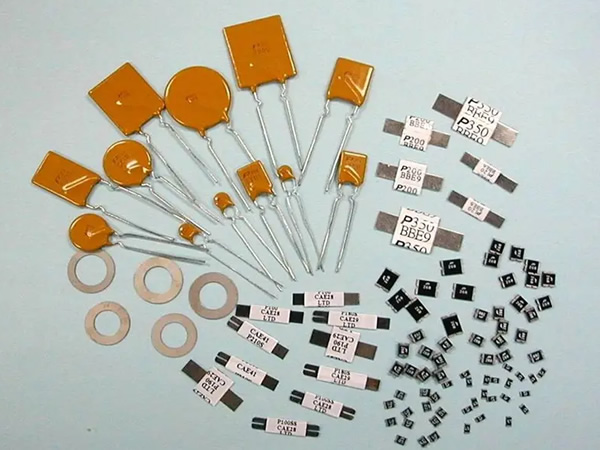### What Are Thermistors And Their Main Types And Parameters?Thermistor - model MZ, MF:
It is a kind of non-linear resistor that is sensitive to temperature response and the resistance value will change with the change of temperature. It is usually made of single crystal and polycrystalline semiconductor materials.
Text symbol: "RT" or "R"
Type of thermistor:
A. According to the structure and shape, there are many kinds of thermistors, such as circular (sheet), cylindrical (cylindrical), circular (gasket) and so on.
B. According to the sensitivity classification of temperature change - high sensitivity (sudden change) and low sensitivity (slow change) thermistors.
C. Classified by heating mode - direct heating thermistor and side heating thermistor.
D. According to the characteristics of temperature change (temperature change) - positive temperature coefficient (PTC), negative positive temperature coefficient (NTC) thermistors.

Main parameters of thermistor: in addition to the basic indicators such as nominal resistance, rated power and allowable deviation, there are also the following indicators:
1) Measuring power: refers to the power consumed when the resistance value of the resistor is not more than 0.1% due to the heating of the measuring power supply under the specified ambient temperature.
2) Material constant: it is the index of thermal sensitivity of reaction thermistor. Generally, the higher the value, the higher the sensitivity and resistivity of the thermistor.
3) Resistance temperature coefficient: refers to the relative change of resistance value caused by each 1 ℃ temperature change of thermistor under zero power condition.
4) Thermal time constant: refers to the thermal inertia of the thermistor. That is, in the reactive power state, when the ambient temperature changes suddenly, the time required for the resistance body temperature to change from the initial value to 63.2% of the difference between the final temperature.
5) Dissipation coefficient: refers to the power dissipated by each 1 ℃ increase in the temperature of the thermistor.
6) Switch temperature: refers to the temperature when the zero power resistance value of the thermistor is twice the lowest resistance value.
7) Maximum operating temperature: refers to the maximum temperature that the thermistor is allowed to bear when it works continuously for a long time under the specified standard conditions.
8) Nominal voltage: refers to the voltage value corresponding to the nominal working current of the thermistor for voltage stabilization under the specified temperature
9) Working current: refers to the specified current value of the thermistor for voltage stabilization under normal working condition.
10) Voltage stabilization range: refers to the range value of the voltage stabilized by the thermistor within the specified ambient temperature range.
11) Maximum voltage: refers to the maximum voltage value allowed to be continuously applied when the thermistor works normally under the specified ambient temperature.
12) Insulation resistance: refers to the resistance value between the resistance body of the thermistor and the insulation shell under the specified environmental conditions.

● positive temperature coefficient thermistor (PTC)

Structure - made of barium titanate (BaTiO3), strontium (SR), zirconium (Zr) and other materials.
It is a direct heating thermistor.
Characteristics - the resistance value is directly proportional to the temperature change, that is, when the temperature rises, the resistance value will increase. At room temperature, its resistance value is small, only a few ohms to several tens of ohms; When the current flowing through it exceeds the rated value, its resistance value can rapidly increase to hundreds of ohms to thousands of ohms within a few seconds. Function and application: it is widely used in the degaussing circuit of color TV, the starting circuit of refrigerator compressor and the circuit of overheating or overcurrent protection, and can also be used in small household appliances such as electric mosquito repellents and hair curlers, electric heating pads and heaters.

● negative temperature coefficient thermistor (NTC)

Structure - made of metal oxides (with semiconductor properties) such as manganese (MN), cobalt (CO), nickel (Ni), copper (Cu), aluminum (AL) or silicon carbide (SIC) by ceramic process.
Characteristic - the resistance value is inversely proportional to the temperature change, that is, when the temperature rises, the resistance value decreases.
Function and application: it is widely used in household appliances and office products such as refrigerators, air conditioners, microwave ovens, electric ovens, photocopiers, printers, etc., for temperature detection, temperature compensation, temperature control, microwave power measurement and voltage stabilization control.
Let's Start
A New Project Today.